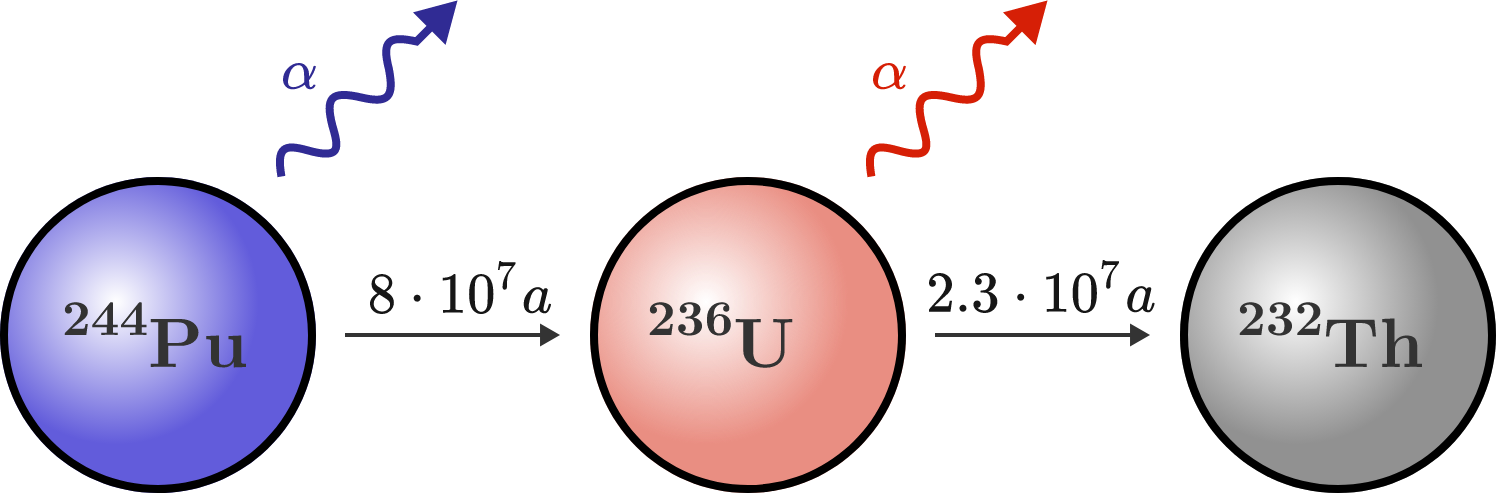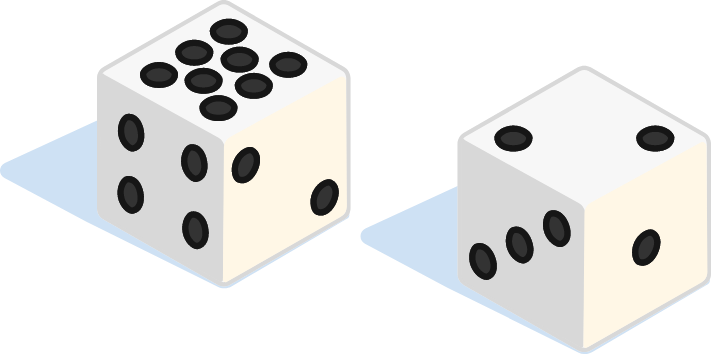# Problems of the Week

Contribute a problem

Kyle draws 5 cards from a standard deck of cards. The probability that he has a flush (including straight and royal flushes) is $\frac{ 4 \times { 13 \choose 5 } } { 52 \choose 5 } = \frac{ 33} { 16660 }.$ From the remaining 47 cards, Linh draws 5 cards. Given that Kyle has a flush, what is the probability that Linh has a flush?Kyle hosts a DataSkeptic podcast, which explores topics in statistics, machine learning, big data, artificial intelligence, and data science. He focuses on scientific skepticism, misconceptions, and misinformation in these topics.

How many positive integer solutions $(a, b)$ does the equation $a^2+b^2+(a+b)^2=b^3$ have, where $0Going up! You get into a stationary elevator with a bouncing ball. Its collisions with the floor are perfectly elastic; it always bounces to the same height—in an inertial frame. In this problem, we will simulate what happens to the bounce as the elevator’s acceleration increases very slowly over time.

Suppose at $t=0$ the height of the ball's bounce is $h_0 = \SI{1}{\meter}$ and the elevator begins accelerating upward with a slowly increasing rate $a(t) = \epsilon t.$ When the acceleration of the elevator reaches $a=\frac12 g,$ what is the peak height of the ball above of the floor in each bounce?

Details & Assumptions

• $\epsilon=\SI[per-mode=symbol]{0.01}{\meter\per\second\cubed}.$
• Assume the acceleration due to gravity is $g=\SI[per-mode=symbol]{10}{\meter\per\second\squared}$ everywhere.
• The elastic bounces are instantaneous so that the upward speed of the ball after the bounce is $v_\text{after} = 2 v_\text{elev}-v_\text{before}$ where $v_\text{elev}$ is the instantaneous speed of the elevator. $v_\text{before}$ is the downward velocity of the ball, and $v_\text{before} \lt 0.$
• The instantaneous collisions cannot be described easily using a time-stepping method. Simulate the bouncing exactly to avoid inaccuracies accumulated over many simulated bounces.

import math

g = 10.0 #gravity
epsilon = 0.01 #rate of increase of acceleration

#Initially, the ball is coming off a bounce with velocity that would take it to a height of 1 m in a stationary elevator.
initialState = {"vBall": math.sqrt(2 * g), "vElev": 0, "aElev":0} #vBall: ball velocity // vElev: elevator velocity // aElev: elevator acceleration

def update(state):
# This function uses kinematics to calculate exactly how much time elapses between two consecutive bounces.
# Then computes vBall, vElev and aElev immediately after the next bounce.
#
#Input: the state of the system as the ball is coming off a bounce with the elevator floor
#Returns: the state immediately after the next bounce. Calling this function repeatedly moves the system forward in time.

###Add code HERE to compute the state of the ball immediately before the next bounce###

beforeCollision = {"vBall": vBall_New, "vElev": vElev_New, "aElev": aElev_New} #state right before the collision with the floor
afterCollision = bounce(beforeCollision) #apply the effect of the collision on vBall
return afterCollision

def bounce(state):
#This function applies the update rule to vBall during an instantaneous elastic collision with the floor.
return {"vBall": (-state["vBall"] + 2 * state["vElev"]), "vElev": state["vElev"], "aElev": state["aElev"]}

def hmax(state):
#This function computes the maximum height after a bounce. At tmax, the relative speed vBall-vElev = 0 and the ball reaches its max height.
tmax = 1 / (epsilon) * (-(g + state["aElev"]) + math.sqrt((g + state["aElev"])**2 + 2 * epsilon * (state["vBall"] - state["vElev"])))

#Returns the difference between the height of the ball and the elevator floor
return state["vBall"] * tmax - 0.5 * (g + state["aElev"]) * tmax ** 2 - state["vElev"] * tmax - 1/6 * epsilon * tmax**3

def simulate(state):
#This function updates the initial state until the acceleration exceeds g/2, then prints the max height of the previous bounce.
while (state["aElev"] < 0.5 * g):
prevState = state #Save the previous state of the system
state = update(state) #Compute the state after the next bounce

print("The max height is %f" % hmax(prevState))

simulate(initialState)
Python 3
You need to be connected to run code

NASA has captured an extraterrestrial space probe that has been in space for millions of years. The probe was operated by a kind of radionuclide battery, which on launch consisted of a pure block of plutonium-244. Now, the isotope ratio between thorium-232 and plutonium-244 is 1:1 due to the radioactive decay $(N_\text{Pu} = N_\text{Th}).$

How long was the probe in space? (Specify the time in millions of years and round it to the nearest integer.)Details and Assumptions:

• Plutonium-244 decays in a two-step reaction into thorium-232: $^{244}\text{Pu} \longrightarrow \,^{236}\text{U} \longrightarrow \,^{232}\text{Th}.$
• The half-lives of plutonium-244 and uranium-236 are 80 and 23 million years, respectively.
• Thorium-232 is stable (half-life is longer than 10 billion years).

I have two distinct, fair, six-sided dice that have positive integers on their sides. Neither of the dice is a "normal" die (i.e., with 1, 2, 3, 4, 5, and 6 on the sides).

Interestingly, the probability distribution of the sum of rolling these two dice is exactly the same as that of the sum of rolling 2 "normal" dice.

Let the numbers on the first die be $(a_1, a_2, a_3, a_4, a_5, a_6),$ and those on the second $(b_1, b_2, b_3, b_4, b_5, b_6),$ where $a_1\leq a_2 \leq\cdots\leq a_6$ and $b_1\leq b_2 \leq \cdots\leq b_6.$

Submit your answer as the product of these two 6-digit integers: $\overline{a_1 a_2 a_3 a_4 a_5 a_6} \times \overline{b_1 b_2 b_3 b_4 b_5 b_6 }.$The dice above have 1,1,2,2,4,8 and 1,2,3,3,4,4 on their sides. Their sum can produce the same numbers (2-12) as the sum of two ordinary dice, but not with the same probability distribution.

×